Violating Anna Karenina Principle: LogWorth scaling

The first line of Leo Tolstoy’s classic novel, Anna Karenina, begins:

“Happy families are all alike; every unhappy family is unhappy in its own way.”

This is a very memorable line. But looking at the Wikipedia entry for the Anna Karenina Principle, I saw this version for statistical significance tests:

“… there are any number of ways in which a dataset may violate the null hypothesis and only one in which all the assumptions are satisfied.”

When we do a hypothesis test that two means are the same, the boring case is where they are the same, i.e., where we do not reject the null hypothesis. The interesting case is where the means are different, though perhaps not interesting enough to be worth a Tolstoy novel. The difference can be enough to be significant. The difference can be many times the difference that would be significant. They can be different in one direction or the other. When we have more than two means, there are lots of combinations of differences that are of interest. We are looking for something interesting – something different – in the statistics.

Consider a manufacturing process where you want to look for differences. Suppose you changed a supplier for one ingredient, and you want to make sure that the process or product doesn’t change, or changes in the right direction. Suppose you make an engineering change in how the product is made, and you want to make sure it doesn’t get worse. You have hundreds of variables to look at, and you want to test hundreds of hypotheses. You want to see where the biggest differences are.

Let’s look at some semiconductor data. We want to keep an eye on 387 process variables, and we are worried about an engineering change, described in the column “Process” as Old or New. I can easily do a Fit Y by X for all the responses versus Process. Then I do a control-click on ANOVA to get all the tests. The control-click broadcasts the command to all 387 analyses. Here is a picture of one of the 387 reports: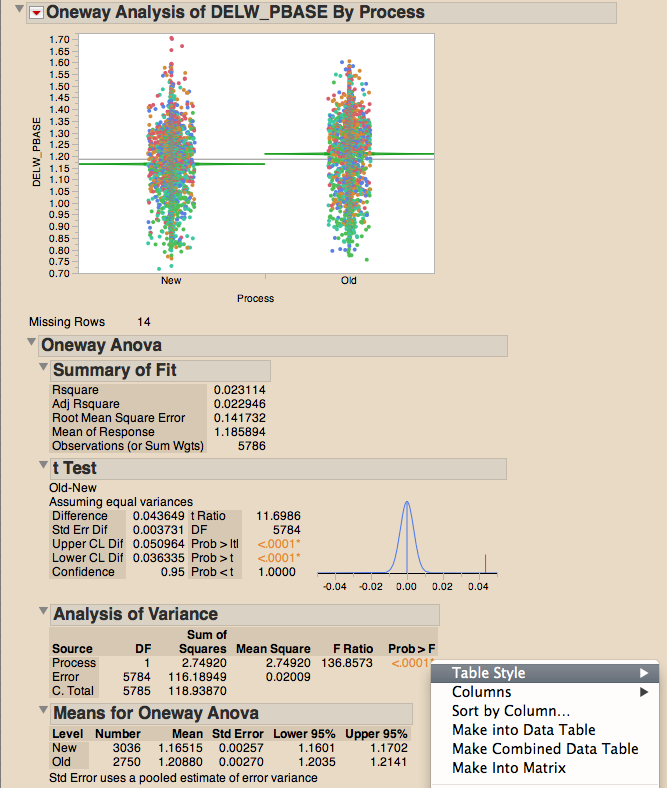Now I have the same problem as described in my previous blog post: There are 387 reports to look through. I just want to look at the ANOVA p-value, “Prob > F”, the probability of getting an even greater F statistic than I got, given the means are equal. Well, JMP can gather these p-values. I right-click on the value and choose the menu item “Make Combined Data Table,” resulting in this: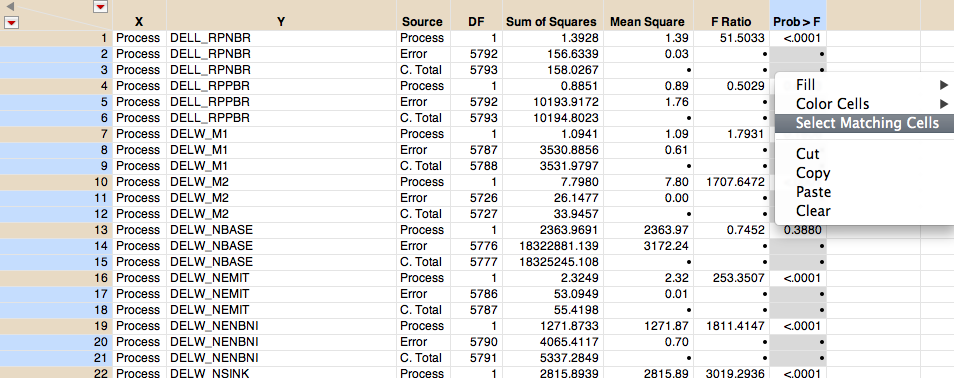The table has three lines per response in it, but the rows I want to delete all have missing values for the p-value. So I can use Select Matching Cells to select them, delete them and then sort them in ascending order by another right click command in the p-value column.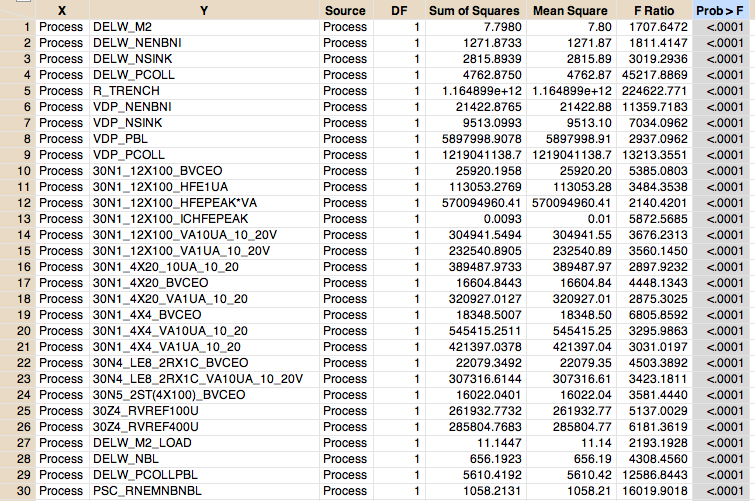Hey! It looks like many of my p-values are significant. Something really did change when I changed the process. Here is the distribution of p-values: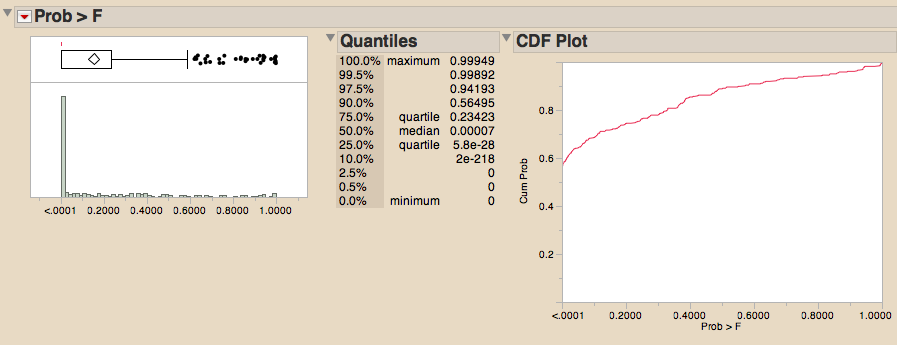Looking at the CDF plot, I see that almost 60 percent of the p-values are very small. The other 40% of the p-values seem fairly uniformly distributed from 0 to 1. Look at all the p-values in order, small to large: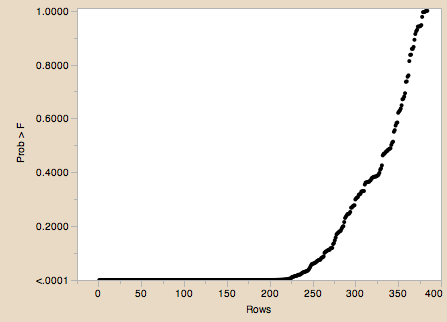Remember the Anna Karenina Principle now. When the means are the same, i.e., the null hypothesis is not rejected, it is all boring sameness. But when the means are different, the null hypothesis is rejected, and the means can be different in many ways, in many magnitudes. Visually, I want sameness to look like sameness, and I want significant differences to look different and interesting.

In a p-value plot, we have the reverse: For all the non-interesting tests where the means are the same, the p-values are easily distinguishable. For all the interesting tests where the means are all different in interesting ways, they all have values close to zero where I can’t tell one from another. P-values violate the visual Anna Karenina principle – you can’t see the interesting, but you can see the boring. It is as if Tolstoy wrote many chapters on the dull peripheral characters, and only a few lines on the main characters in the novel. We need to fix this.

The obvious way to get detail for very small positive values is to switch to the log scale. It turns out that I have to pick a very small origin to get most of these p-values.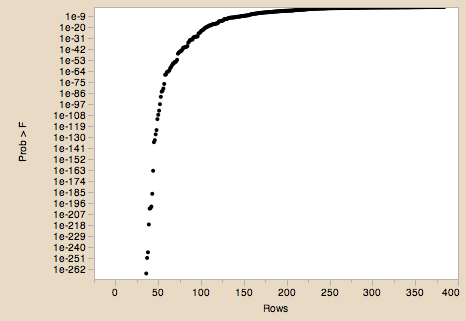Rather than just using a log scale, why not just calculate log10(pvalue) and plot that? In fact, we would like to have the most significant values on top, not bottom, so we flip signs and plot –log10(pvalue). And because we want to do this a lot, we need to invent a short name for this, so let’s call it LogWorth.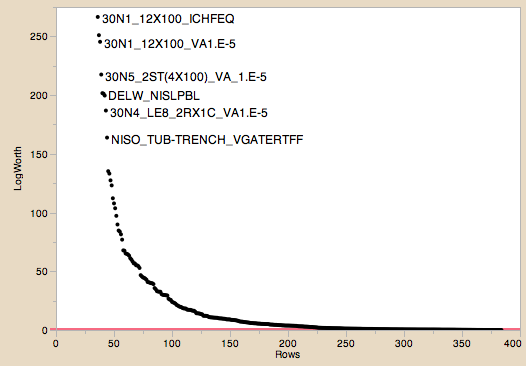The name LogWorth was used starting in 1995 by Padraic Neville in the specific context of decision tree criteria. It is a lot easier to say “LogWorth” than to say “negative log-to-the-base-10 of the p-value.”

So now we have come into compliance with the Anna Karenina Principle. Sets of means that are significantly different are different in the significance plot. Means that are boringly the same are the same in the plot.

But have we given up some perspective? Will we understand LogWorth as well as probabilities? I am optimistic that we can. Here are the new goalposts of significance to learn:

PValue = .01 -> LogWorth = 2

PValue = .05 -> LogWorth = 1.3

PValue = .10 -> LogWorth = 1

In the plot above, I even have a red reference line at 2. Also we are comfortable talking about information on the log probability scale, as you can envision with phrases like “as rare as three 10s in a row in 10-sided die tosses,” which is (1/10)3 or.001.

Information theory is very comfortable with the log-scale, where you can add instead of multiply. The units terminology has been figured out. Negative log to the base 2 of probability in information theory is called bits; if you use the base 10, it is called decits. So we can see that the LogWorth axis is measured in decits of rareness, or how many decahedron die rolls. (For several alternative terms, see the Units of information entry in Wikipedia.)

The log scale is especially important when handling tests that are extremely significant, which happens all the time with large data sets. You still want to plot and rank significance. But the floating point system of our computers can only represent values down to about 10^-323. In our data, of the 387 tests we have done, 38 of the tests have p-values smaller than 10^-323, representable only as zero, and thus not distinguishable. We therefore use log-probability routines whenever we calculate significances, so that we can keep representing significance. The most significant test in this data has a LogWorth of 4638.1808456. That is a very distinguished value, so much that it distorts a plot of the LogWorths – in some cases we may need to either use another log transform. Actually, we will put a ceiling on the LogWorth – in the interest of seeing more details in the rest of the data, and apologizing for violating the visual Anna Karenina Principle for a few values.

By the way, LogWorths started appearing the Partition platform at its introduction, and now the Response Screening platform and associated fitting personality use it throughout.

Note: This is part of a Big Statistics series of blog posts by John Sall. Read all of his Big Statistics posts.

Article Labels

There are no labels assigned to this post.

Article Tags
1 Comment
Visitor

Kayle Sawyer wrote:

Using -log10(pvalue) is standard practice with some neuroimaging packages (e.g. FreeSurfer). It is especially useful when creating statistical spatial maps of multiple adjacent comparisons. It would be great if this term, "LogWorth," caught on!